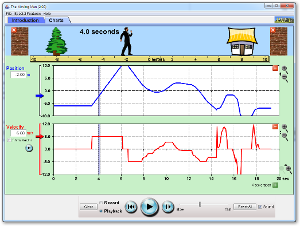Now let's look at how to graph constant acceleration. In this section, you will complete a lab simulation studying constant acceleration graphs. You will analyze data collected using real-time equipment such as motion detectors or photogates to determine the motion of an object.

Click on the Charts tab at the top. Delete the top graph by clicking on the “-“button in the upper right hand corner and delete the building. Create the following scenarios by adjusting the velocity and acceleration amount or by moving the arrows. Create a sketch of each graph.

Try various scenarios such as the following:

1. Zero initial velocity with a positive acceleration
2. Positive initial velocity with a positive acceleration
3. Positive initial velocity with a negative acceleration
4. Positive initial velocity with zero acceleration

Click on the link to access the applet. Pay attention to how the graphs change as you work through various scenarios. Also, pay attention to how the velocity and acceleration vector changes.Click to Run